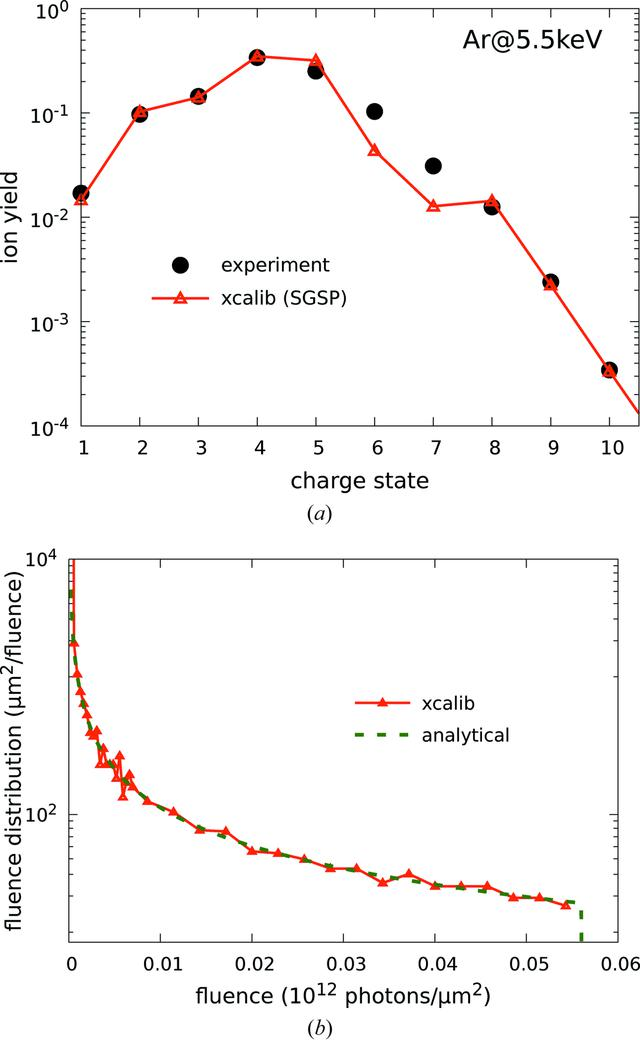disable zoom     view article Figure 5 (a) Ar CSD at 5.5 keV by Fukuzawa et al. (2013) is shown. The black line with dots shows the experimental results. The red line with triangles shows the theoretical results obtained by xcalib with the SGSP, equation (22). (b) Numerical and analytical FDFs for the SGSP, equation (22). The analytical formula is given by equation (42).JOURNAL OFSYNCHROTRONRADIATION
ISSN: 1600-5775
Volume 26| Part 4| July 2019| Pages 1017-1030